## Unit 1

Open
Unit 1 Lesson 1 (04/01/2021 – 04/01/2022)
1
Worksheet
Level 1 – Unit 1 – Lesson 1 (Worksheet 2)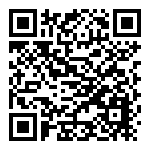2
Curriculum Card
Level 1 – Unit 1 – Lesson 1 (Worksheet 2)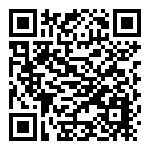3
Tutorial
Level 1 – Unit 1 – Lesson 1 (Worksheet 2)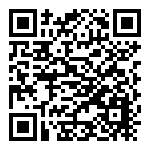4
Editable Flashcards
Level 1 – Unit 1 – Lesson 1 (Worksheet 2)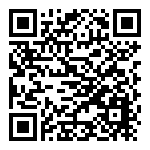5
ABC EGGSplorers
Level 1 – Unit 1 – Lesson 1 (Worksheet 2)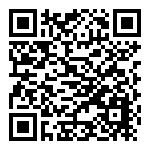6
Level 1 – Unit 1 – Lesson 1 (Worksheet 2)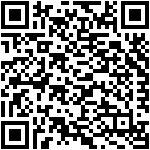This QR Code list was made using the BINGOBONGO FUN!box
Open
Unit 1 Lesson 1 (04/01/2021 – 04/01/2022)
1
Worksheet
Level 1 – Unit 1 – Lesson 1 (Worksheet 2)2
Curriculum Card
Level 1 – Unit 1 – Lesson 1 (Worksheet 2)3
Tutorial
Level 1 – Unit 1 – Lesson 1 (Worksheet 2)4
Editable Flashcards
Level 1 – Unit 1 – Lesson 1 (Worksheet 2)5
ABC EGGSplorers
Level 1 – Unit 1 – Lesson 1 (Worksheet 2)6
Level 1 – Unit 1 – Lesson 1 (Worksheet 2)This QR Code list was made using the BINGOBONGO FUN!box
Open
Unit 1 Lesson 1 (04/01/2021 – 04/01/2022)
1
Worksheet
Level 1 – Unit 1 – Lesson 1 (Worksheet 2)2
Curriculum Card
Level 1 – Unit 1 – Lesson 1 (Worksheet 2)3
Tutorial
Level 1 – Unit 1 – Lesson 1 (Worksheet 2)4
Editable Flashcards
Level 1 – Unit 1 – Lesson 1 (Worksheet 2)5
ABC EGGSplorers
Level 1 – Unit 1 – Lesson 1 (Worksheet 2)6
Level 1 – Unit 1 – Lesson 1 (Worksheet 2)This QR Code list was made using the BINGOBONGO FUN!box
Open
Unit 1 Lesson 1 (04/01/2021 – 04/01/2022)
1
Worksheet
Level 1 – Unit 1 – Lesson 1 (Worksheet 2)2
Curriculum Card
Level 1 – Unit 1 – Lesson 1 (Worksheet 2)3
Tutorial
Level 1 – Unit 1 – Lesson 1 (Worksheet 2)4
Editable Flashcards
Level 1 – Unit 1 – Lesson 1 (Worksheet 2)5
ABC EGGSplorers
Level 1 – Unit 1 – Lesson 1 (Worksheet 2)6